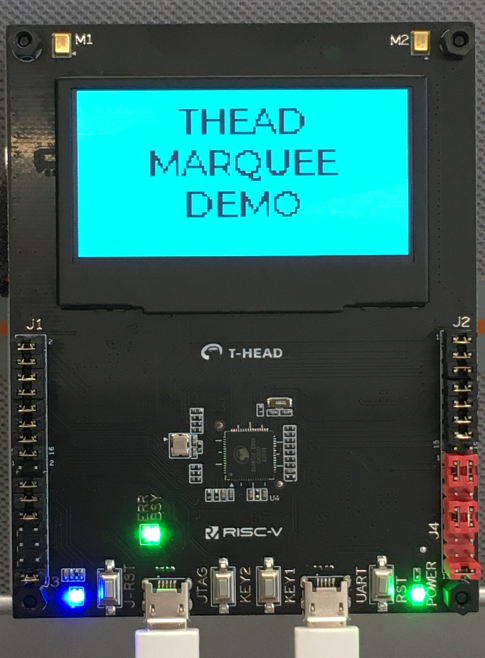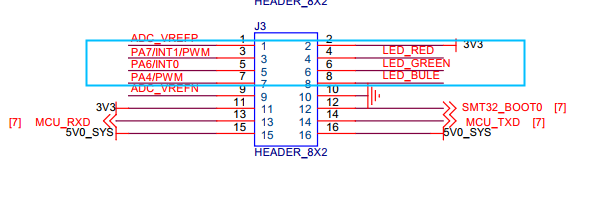# RVB2601 应用开发实战系列二: 跑马灯## 2. 硬件配置## 3. 跑马灯开发

### 3.1 例程下载### 3.2 LED 配置

• led_pinmux_init

void led_pinmux_init(){        //7    csi_pin_set_mux(PA7, PA7_PWM_CH7);    csi_pin_set_mux(PA25, PA25_PWM_CH2);    csi_pin_set_mux(PA4, PA4_PWM_CH4);    ret = csi_pwm_init(&r, 0);    ret = csi_pwm_out_config(&r, 7 / 2, 300, 100, PWM_POLARITY_HIGH);    ret = csi_pwm_out_start(&r, 7 / 2);        //25    ret = csi_pwm_out_config(&r, 2 / 2, 300, 100, PWM_POLARITY_HIGH);    ret = csi_pwm_out_start(&r, 2 / 2);        //4    ret = csi_pwm_out_config(&r, 4 / 2, 300, 100, PWM_POLARITY_HIGH);    ret = csi_pwm_out_start(&r, 4 / 2);}

• led_refresh

void led_refresh(){    g_ctr++;    if (g_ctr == 3) {            g_ctr = 0;    }    if (g_ctr == 0)    {        csi_pwm_out_start(&r, 7 / 2);        csi_pwm_out_stop(&r, 2 / 2);        csi_pwm_out_stop(&r, 4 / 2);    }    else if (g_ctr == 1)    {                csi_pwm_out_start(&r, 2 / 2);        csi_pwm_out_stop(&r, 7 / 2);        csi_pwm_out_stop(&r, 4 / 2);    }    else //2    {        csi_pwm_out_start(&r, 4 / 2);        csi_pwm_out_stop(&r, 7 / 2);        csi_pwm_out_stop(&r, 2 / 2);    }}

static void demo_task(void *arg){    lv_init();    oled_init();    lable_test();    led_pinmux_init();    while (1)    {        lv_task_handler();        udelay(1000 * 1000);        lv_tick_inc(1);        led_refresh();    }}

### 3.3. 编译运行## 4. 总结## 评论